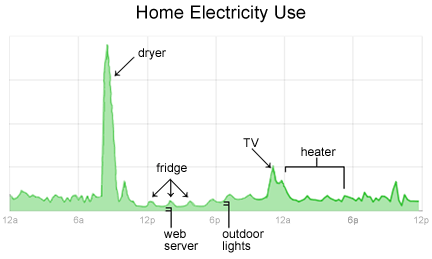Search IntMath
Close

450+ Math Lessons written by Math Professors and Teachers

5 Million+ Students Helped Each Year

1200+ Articles Written by Math Educators and Enthusiasts

Simplifying and Teaching Math for Over 23 Years

# Energy reduction with Google’s help

By Murray Bourne, 23 Feb 2009

Google.org describes how the use of "smart meters" can reduce energy consumption.

If you know how much you use each day in real time, it can make a significant change to the way you use electricity without necessarily making a dent in your lifestyle.Google outlines some sources and calculations and concludes:

Based on these figures, we get an average of 7.5 tonnes of CO2 emitted per year per household, and 4.5 tonnes of CO2 emitted per year per conventional car. So, 10% energy savings for 6 households would reduce carbon emissions by about the same amount as taking one conventional car off the road.

Google's chart and the smart meters will only be available to selected US consumers, but I hope other countries follow suit.

This is an issue that schools (especially math classes) should investigate. Are Google's calculations valid? How can this reduce energy consumption in your school — and home?

Be the first to comment below.

### Comment Preview

HTML: You can use simple tags like <b>, <a href="...">, etc.

To enter math, you can can either:

1. Use simple calculator-like input in the following format (surround your math in backticks, or qq on tablet or phone):
a^2 = sqrt(b^2 + c^2)
(See more on ASCIIMath syntax); or
2. Use simple LaTeX in the following format. Surround your math with $$ and $$.
$$\int g dx = \sqrt{\frac{a}{b}}$$
(This is standard simple LaTeX.)

NOTE: You can mix both types of math entry in your comment.